## The characteristics of volatile

When we declare shared variables to be volatile, read / write will be very special for this variable. A good way to understand the volatile characteristics are: a single reading of the volatile variables / write, using the same monitor as a lock for the single read / write operation done in sync. Let illustrated by specific examples, see the following sample code:

``````class VolatileFeaturesExample {
volatile long vl = 0L;  // 使用 volatile 声明 64 位的 long 型变量

public void set(long l) {
vl = l;   // 单个 volatile 变量的写
}

public void getAndIncrement () {
vl++;    // 复合（多个）volatile 变量的读 / 写
}

public long get() {
return vl;   // 单个 volatile 变量的读
}
}``````

Suppose there are multiple threads call the three methods above program, this program is semantically equivalent and the following procedures:

``````class VolatileFeaturesExample {
long vl = 0L;               // 64 位的 long 型普通变量

public synchronized void set(long l) {     // 对单个的普通 变量的写用同一个监视器同步
vl = l;
}

public void getAndIncrement () { // 普通方法调用
long temp = get();           // 调用已同步的读方法
temp += 1L;                  // 普通写操作
set(temp);                   // 调用已同步的写方法
}
public synchronized long get() {
// 对单个的普通变量的读用同一个监视器同步
return vl;
}
}``````

As shown, a single read example above a volatile variable program / write operations, and a general reading of variable / write operations use the same monitor lock to synchronize, the same effect therebetween.

happens-before guarantees regular monitor lock release and access to memory monitor visibility between the two threads of the monitor, which means reading of a volatile variable, always able to see (any thread) of this volatile variable final written.

Monitor lock semantics determines the execution of a critical section of code atomic. This means that even a 64-bit long and double-type variable, as long as it is a volatile variable, the variable will be read atomic. If a plurality of operations or similar volatile ++ volatile compound such operations, which do not have atomic whole.

In short, volatile variable itself has the following characteristics:

• Visibility. Reading of a volatile variable, always able to see (any thread) last write this volatile variable.
• Atomicity: reading of any single variable volatile / write atomic, but similar to such composite operations are not volatile ++ atomic.

## volatile write - happens before the establishment of the relationship between reading

Mentioned above is volatile variable own characteristics, the programmer, the impact of volatile memory visibility thread is more important than the volatile own characteristics, but also need our attention.

Starting JSR-133, volatile variable write - read communication between threads can achieve.

From memory semantic point of view, with the monitor lock volatile have the same effect: the release of volatile write and monitor have the same semantic memory; read the monitor acquiring volatile memory has the same semantics.

See sample code below volatile variables:

``````class VolatileExample {
int a = 0;
volatile boolean flag = false;

public void writer() {
a = 1;                   //1
flag = true;               //2
}

if (flag) {                //3
int i =  a;           //4
……
}
}
}``````

After thread A execution is assumed writer () method, the thread B executed Reader () method. According happens before the rules, a process that happens before the establishment of the relationship can be divided into two categories:

1. The program sequence rules, 1 happens before 2; 3 happens before 4.
2. The volatile rule, 2 happens before 3.
3. The transfer happens before the rules, 1 happens before 4.

The patterned manifestation before the relationship happens as follows: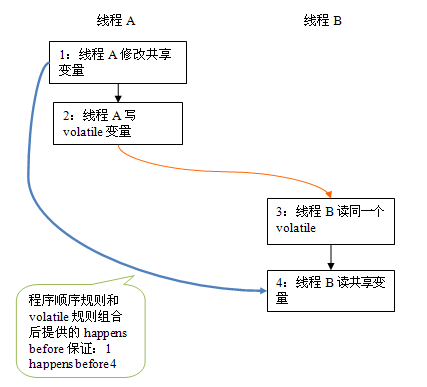In the figure, the two nodes of each link of the arrow, represents a happens before relationship. Black arrows program sequence rules; orange arrows indicate volatile rule; blue arrows indicate happens before the rules to ensure that the composition provide.

A thread here after writing a volatile variable, B threads read the same volatile variable. A thread before writing volatile variables all visible shared variables, thread B after reading the same volatile variable, will immediately become visible to thread B.

## volatile write - read semantic memory

write volatile memory semantics are as follows:

• When writing a volatile variable, JMM will the thread corresponding local memory shared variables flushed to main memory.

In the above example program VolatileExample example, assume that first executes the thread A writer () method, then thread B executed Reader () method, the initial two thread local memory and a flag are in an initial state. The figure is executed after the thread A volatile writing, the state of a shared variable schematic: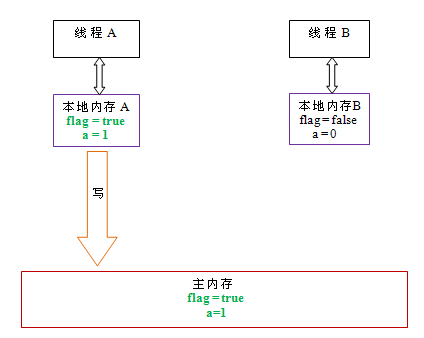As shown above, after writing the thread A flag variable, local memory A thread values ​​are updated two A shared variable is flushed to main memory. At this time, the value of local memory and the main memory A shared variable are consistent.

volatile memory semantics to read as follows:

• When reading a volatile variable, JMM will the thread corresponding local memory is deasserted. Then the main thread read shared variables from memory.

The following is the thread B reads the same volatile variable, a schematic view of the status of shared variables: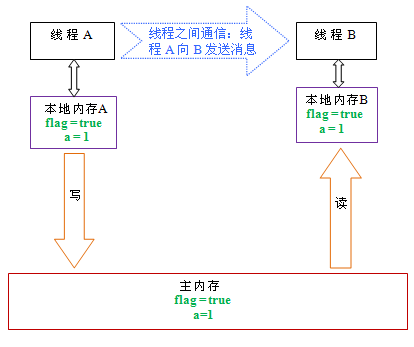As shown above, after reading flag variable, local memory B has been deasserted. At this time, the thread B must be read from main memory shared variables. Reading operation thread B will cause the value B and the local memory shared variables in main memory also becomes the same.

If we volatile write and volatile read these two steps together to see if, after reading the thread B reads a volatile variable, write thread A before writing this volatile variable all visible values ​​of shared variables will immediately become to read threads B visible.

Next, volatile and volatile read-write memory semantics to be a summary:

• A thread write a volatile variable, is essentially a thread A issued (where modification of its shared variable) to the next message will be read this volatile variables a thread.
• A thread is a volatile variable to write, then thread B reads the volatile variables, the process is essentially the thread A sends a message to the thread B through the main memory.

## volatile memory implementation semantic

Now, let's look at how semantic memory JMM to achieve volatile write / read.

We mentioned previously compiled into the reordering and sorting discouraged processor reordering. In order to achieve volatile memory semantics, JMM limit the types of reordering both types, respectively. The following is a compiler developed for the JMM volatile reordering rules table:

 Can reordering The second operation The first operation Normal read / write volatile read volatile write Normal read / write NO volatile read NO NO NO volatile write NO NO

For example, the third line of the last cell means: in program order, when the first operation is a read or write simple variables, if the second write operation as volatile, the compiler can not reorder this two operations.

From the table we can see:

• When the second operation is a volatile time of writing, no matter what is the first operation that can not be reordered. This rule ensures that the volatile write operation before will not be compiled thinks highly volatile after ordering to write.
• When the first operation is a volatile read when, no matter what is the second operation, it can not be reordered. This rule ensures that volatile read operation will not be compiled after ordering discouraged to read before volatile.
• When the first write operation is a volatile, volatile second operation is a read, no reordering.

In order to achieve volatile memory semantics, the compiler when generating bytecode will be inserted in the instruction sequence memory barrier to inhibit a particular type of processor reordering. For the compiler, find an optimal arrangement of the total number of insertion to minimize barriers is almost impossible, therefore, JMM take a conservative strategy. The following is based on a conservative strategy JMM memory barrier insertion strategies:

• Each preceding write operation to insert a volatile StoreStore barrier.
• Write back operation is inserted in each of a volatile StoreLoad barrier.

Insert memory barrier above strategy is very conservative, but it can ensure that any processor platform, any program can get correct volatile memory semantics.

The following is a conservative strategy, volatile schematic write command sequence generated after inserting memory barriers: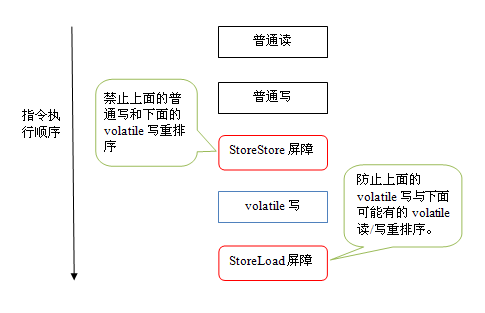The figure above StoreStore barrier can be guaranteed before the volatile write front of all ordinary write operation has to be seen in any of the processor. This is because StoreStore barrier will protect all of the above general write flushed to main memory before the volatile write.

The following is the conservative strategy, volatile read instruction memory barriers generated after insertion sequence diagram: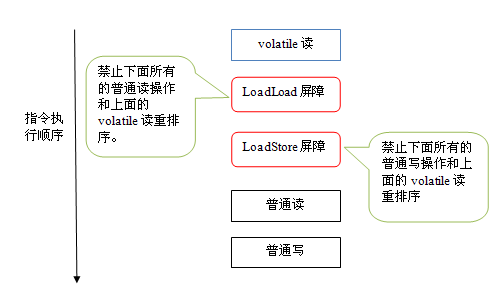Figure above LoadLoad barrier to disable the processor to read the above and below normal volatile read reordering. LoadStore barrier to disable the processor to read the above and below normal volatile write reordering.

Above volatile and volatile read-write memory barrier insertion strategy is very conservative. In the actual implementation, without altering the volatile write - read memory semantics, the compiler unnecessary barrier may be omitted depending on the circumstances. Below we describe a specific example of the code by:

``````class VolatileBarrierExample {
int a;
volatile int v1 = 1;
volatile int v2 = 2;

int i = v1;           // 第一个 volatile 读
int j = v2;           // 第二个 volatile 读
a = i + j;            // 普通写
v1 = i + 1;          // 第一个 volatile 写
v2 = j * 2;          // 第二个 volatile 写
}

…                    // 其他方法
}``````

For readAndWrite () method, the compiler generates bytecode upon optimization can be done as follows: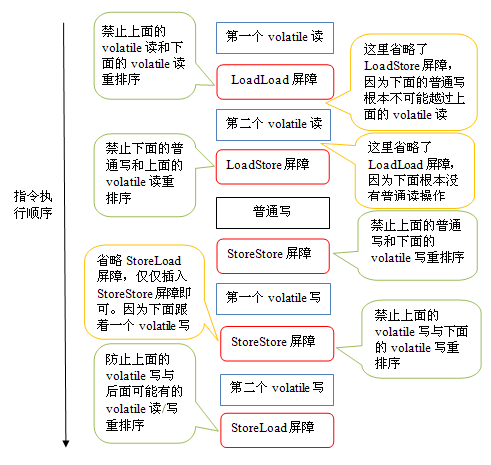Note that the last of StoreLoad barrier can not be omitted. Because after the second volatile write methods return immediately. At this time, the compiler may not accurately determine whether there will be volatile later read or write, for safety reasons, the compiler will often insert here a StoreLoad barrier.

The above optimization was for any processor platform, due to the different processors have different "tightness" in the processor memory model, the memory barrier insert can continue to optimize processor memory according to the specific model. In x86 processors, for example, the image above except for the last barrier StoreLoad other barrier will be omitted.

volatile read and write in front of the conservative strategy, in the x86 processor platform can be optimized to: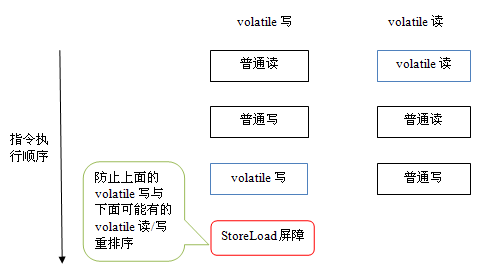Mentioned before, x86 processors will only write - read do reordering. X86 does not read - read, read - write and write - write operations do reordering, so the x86 processors will omit these three operations corresponding to the type of memory barrier. In the x86, JMM only the volatile write back insert a StoreLoad barrier can be realized volatile write correct - read memory semantics. This means that x86 processors, volatile volatile read write overhead than the cost will be much larger (because the execution StoreLoad barrier overhead will be relatively large).

## Why JSR-133 to enhance the volatile memory semantics

In the old JSR-133 Java memory model before, although not allowed reordering between volatile variables, but the old Java memory model allows reordering between volatile and a regular variable. In the old memory model, VolatileExample exemplary program may be reordered to the following sequence is performed: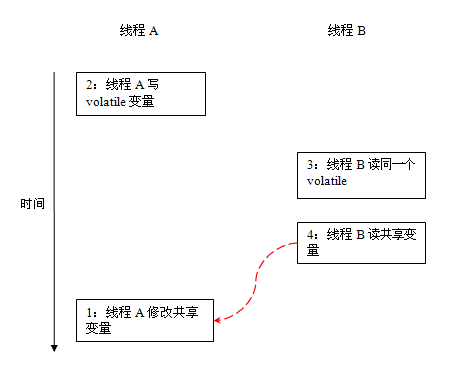In the old memory model, between 1 and 2 when no data dependencies, could be reordered (similar to 3 and 4) between 1 and 2. The result is: Read the thread B executes 4, may not be able to see the writing thread A modification in the implementation of shared variables 1:00.

So in the old memory model, volatile write - read did not release the monitor - won has memory semantics. In order to provide a mechanism for communication between a more lightweight than the monitor lock threads, JSR-133 enhanced the group decided volatile memory semantics: the compiler and processor strictly limited to volatile variables with normal reordering variables to ensure volatile write - read and monitor release - get the same, has the same memory semantics. High regard and collation processor memory barrier insertion strategy compiled from the point of view, as long as the reordering between the volatile variables and common variables may destroy volatile memory semantics, this reordering will be compiled discouraged collation processor and memory barrier insert policy prohibited.

Since volatile read only assures that the individual volatile variables / write atomic property, and the characteristics of the monitor mutex lock executed to ensure the implementation of the entire atomic critical section of code. Functionally, the monitor lock is more powerful than volatile; on the scalability and execution performance, volatile advantage. If readers would like to replace the monitor with volatile program lock, be sure to be cautious.

Published 136 original articles · won praise 6 · views 1487

### Guess you like

Origin blog.csdn.net/weixin_42073629/article/details/104741609
Recommended
Ranking
Daily